﻿ 基于Shearlet变换的自适应地震资料随机噪声压制
 石油地球物理勘探2019, Vol. 54Issue (4): 744-750  DOI: 10.13810/j.cnki.issn.1000-7210.2019.04.0030文章快速检索 高级检索

### 引用本文TONG Siyou, GAO Hang, LIU Rui, CHEN Xueguo. Seismic random noise adaptive suppression based on the Shearlet transform. Oil Geophysical Prospecting, 2019, 54(4): 744-750. DOI: 10.13810/j.cnki.issn.1000-7210.2019.04.003.### 作者简介童思友, 山东省青岛市松岭路238号中国海洋大学海洋地球科学学院, 266100。Email:tsy@ouc.edu.cn

### 文章历史

① 海底科学与探测技术教育部重点实验室, 山东青岛 266100;
② 青岛海洋科学与技术国家实验室海洋矿产资源评价与探测技术功能实验室, 山东青岛 266061;
③ 中国石油化工股份有限公司胜利油田分公司, 山东东营 257100

Seismic random noise adaptive suppression based on the Shearlet transform
TONG Siyou①②, GAO Hang , LIU Rui , CHEN Xueguo
① Key Laboratory of Submarine Geosciences and Prospecting Techniques, Ministry of Education, Qingdao, Shandong 266100, China;
② Laboratory for Marine Mineral Resources, Qingdao National Laboratory for Marine Science and Technology, Qingdao, Shandong 266061, China;
③ Shengli Oilfield Branch Co., SINOPEC, Dongying, Shandong 257100, China
Abstract: Conventional thresholding methods use a unified threshold for all the transform domain coefficients.However for Shearlet transform, signals and noises in all the scales and directions are different, so a global hard threshold has some limits.Aiming at these limits, a random noise suppression is proposed with improved adaptive threshold functions.On the basis of local threshold, the Bayesian threshold is improved to form an adaptive threshold function suitable for the Shearlet transform.A relation between the signal-to-noise ratio (SNR) and threshold functions is built.Different SNRs have different weighting coefficients to adaptively calculate different thresholds.So seismic signals are preserved and the denoising is greatly improved.Tests on model and real data show that the proposed method can effectively suppress random noise and highlight weak signals.
Keywords: Shearlet transform    random noise    multi-scale geometric analysis    signal-to-noise ratio (SNR)    adaptive threshold function
0 引言

Shearlet变换通过对变换系数设定阈值约束分离信噪，其中阈值函数至关重要。目前常用的阈值函数对多尺度、多方向的Shearlet变换局限性很大，会造成噪声压制不彻底或损失有效信号等问题。为此，本文将信噪比与阈值函数有机关联，在不同尺度下基于信噪比约束自适应求取阈值，实现自适应去噪。在保证有效信号不受损失的情况下，恢复被噪声掩盖的弱信号，有效地改善去噪效果。

1 方法原理 1.1 Shearlet变换

Shearlet变换是通过特定形式的合成膨胀仿射系统构造的一种多尺度几何分析工具，是小波变换的高维扩展，具有接近最优的非线性误差逼近性能，数学结构严谨，计算复杂度低。Shearlet变换由拉普拉斯金字塔变换和方向滤波器组构成。设

 $\boldsymbol{A}=\left(\begin{array}{ll}{a} & {0} \\ {0} & {\sqrt{a}}\end{array}\right)$
 $\boldsymbol{B}=\left(\begin{array}{cc}{1} & {-s} \\ {0} & {1}\end{array}\right)$

 $\psi_{j, l, k}(x)=|\operatorname{det} \boldsymbol{A}|^{j/2} \psi\left(\boldsymbol{B}^{l} \boldsymbol{A}^{j} x-k\right)$ (1)

x为自变量。若使ψ满足Parseval框架，则信号函数fL2(R2)的连续Shearlet变换为

 $\mathrm{SH}_{f}(j, k, l)=\left\langle f, \psi_{j, l, k}\right\rangle$ (2)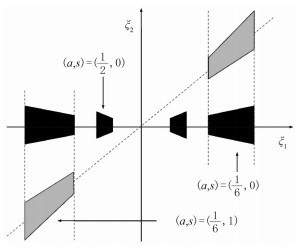图 1 Shearlet基函数频域支撑区间(据文献修改)
1.2 利用Shearlet变换压制随机噪声 1.2.1 Shearlet变换去噪原理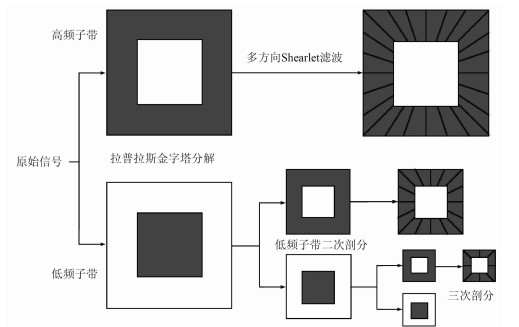图 2 Shearlet变换流程
1.2.2 自适应阈值函数

 $T=\frac{\sigma_\text{n}^{2}}{\sigma_\text{s}}$ (3)

 $T_{\lambda}=\lambda \frac{\sigma_\text{n}^{2}}{\sigma_\text{s}}$ (4)

 $\sigma_{\mathrm{n}}(j, l)=\frac{f_{\mathrm{Median}}(|S(j, l, k)|)}{0.6745}$ (5)

fMedian代表求取中值，σnσs满足σd2=σs2+σn2，且

 $\sigma_{\mathrm{s}}(j, l)=\sqrt{\max \left[\sigma_{\mathrm{d}}^{2}(j, l)-\sigma_{\mathrm{n}}^{2}(j, l), 0\right]}$ (6)
 $\sigma_{\mathrm{d}}^{2}(j, l)=\frac{1}{n} \sum\limits_{k=1}^{n} S^{2}(j, l, k)$ (7)

σd为Shearlet系数的均方根；S(j, l, k)为Shearlet系数；n为某尺度、某方向Shearlet系数的个数。式(4)由不同尺度、不同方向的σnσsλj共同确定，λj与信噪比相关

 $\lambda_{j}=\frac{1}{\operatorname{SNR}(j)}$ (8)

SNR(j)为不同尺度Shearlet系数单独构成的地震信噪比，由相邻地震道互相关求得

 $\operatorname{SNR}(j)=\frac{N \sum\limits_{i=1}^{N-1} Q_{i,i+1}}{(N-1) \sum\limits_{i=1}^{N} Q_{i, i}(0)-N \sum\limits_{i=1}^{N-1} Q_{i, i+1}}$ (9)

 \$ S_{\mathrm{new}}=\left\{\begin{array}{ll}{S} & {|S| \geqslant T_{\lambda}} \\ {0} & {|S|

2 模型试算与实际资料应用 2.1 模型试算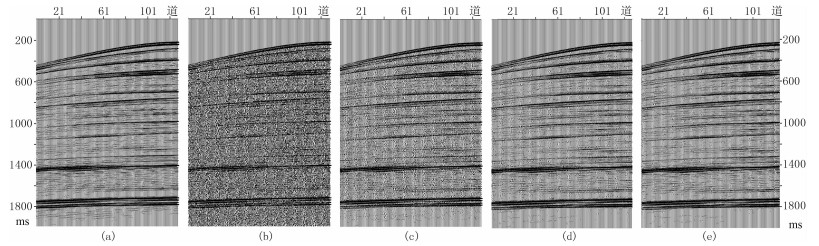图 3 模拟单炮记录去噪效果 (a)模拟单炮记录；(b)图a数据加入高斯噪声；(c)Curvelet变换全局阈值；(d)Shearlet变换全局阈值；(e)本文方法模拟单炮记录共128道，2000个采样点，采样率为1ms。为了更好地呈现去噪效果，进行了顶切处理表 1 不同方法去噪前、后信噪比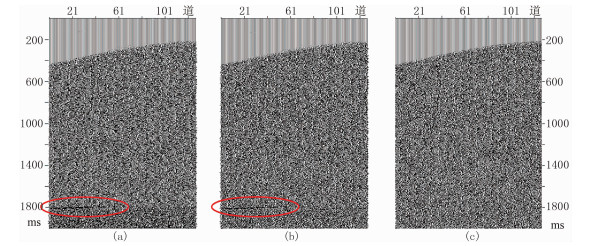图 4 不同方法去噪前、后的残差剖面 (a)图 3c与图 3a数据之差；(b)图 3d与图 3a数据之差；(c)图 3e与图 3a数据之差
2.2 实际资料应用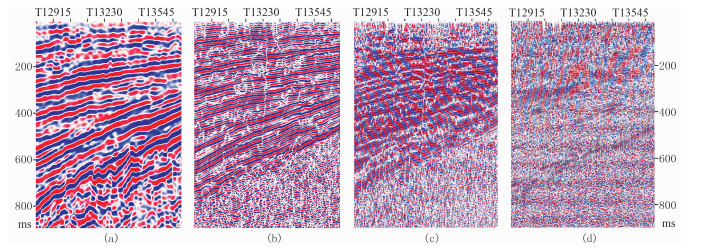图 5 Inline 100629剖面Shearlet变换不同尺度的地震记录 (a)第一尺度；(b)第二尺度；(c)第三尺度；(d)第四尺度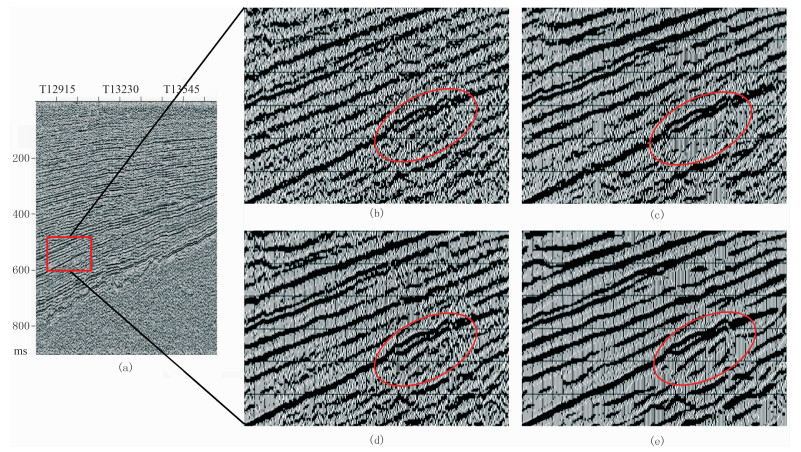图 6 实际地震记录去噪效果 (a)Inline 100629剖面原始记录；(b)图a方框所选数据；(c)Curvelet变换全局阈值；(d)Shearlet变换全局阈值；(e)本文方法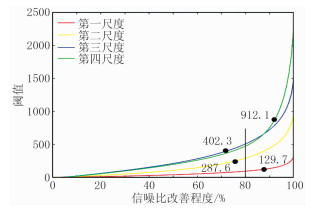图 7 Shearlet变换信噪比改善程度—阈值曲线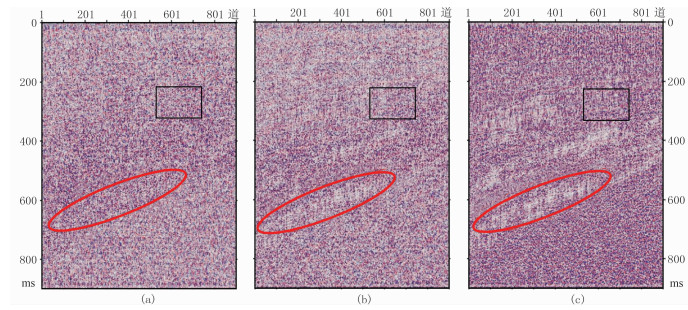图 8 Inline 100629剖面不同方法去噪前、后的残差剖面 (a)Cunelet变换全局阈值；(b)Shearlet变换全局阈值；(c)本文方法表 2 不同区域去噪前、后信噪比
3 结束语

Shearlet变换作为一种多尺度几何分析新方法，较小波变换等传统信号分析方法具有更多优良特性，且运算效率高。另外，针对地震数据去噪过程中传统全局阈值方法的局限性，本文通过改进自适应阈值函数压制随机噪声，在模型试算与实际资料应用中取得了满意的去噪效果，验证了方法的可行性和有效性。

  李庆忠. 走向精确勘探的道路[M]. 北京: 石油工业出版社, 1993.  孔德辉, 彭真明, 江阳.基于广义全变差法的地震信号Shearlet域随机噪声衰减[C].中国石油学会2015年物探技术研讨会论文集, 2015.  康冶, 于承业, 贾卧, 等. f-x域去噪方法研究[J]. 石油地球物理勘探, 2003, 38(2): 136-138. KANG Ye, YU Chengye, JIA Wo, et al. A study on noise-suppression method in f-x domain[J]. Oil Geophysical Prospecting, 2003, 38(2): 136-138. DOI:10.3321/j.issn:1000-7210.2003.02.007  张军华, 陆基孟. 小波变换方法在地震资料去噪和提高分辨率中的应用[J]. 石油大学学报(自然科学版), 1997, 21(1): 18-21. ZHANG Junhua, LU Jimeng. Application of wavelet transform in removing noise and improving resolution of seismic data[J]. Journal of the University of Petroleum (Edition of Natural Science), 1997, 21(1): 18-21.  张军华, 吕宁, 田连玉, 等. 地震资料去噪方法综合评述[J]. 石油地球物理勘探, 2005, 49(增刊): 121-127.  蔡希玲, 吕英梅. 地震数据时频分析与分频处理[J]. 勘探地球物理进展, 2005, 28(4): 265-270. CAI Xiling, LYU Yingmei. Time-frequency analysis and frequency-divisional processing of seismic data[J]. Progress in Exploration Geophysics, 2005, 28(4): 265-270.  Zheng H C, Yu D G, Peng W, et al. A novel fusion algorithm of visible image and infrared image based on NSCT[J]. Advanced Materials Research, 2012, 424-425(1): 223-226.  张华, 陈小宏, 李红星, 等. 曲波变换三维地震数据去噪技术[J]. 石油地球物理勘探, 2017, 52(2): 226-232. ZHANG Hua, CHEN Xiaohong, LI Hongxing, et al. 3D seismic data de-noising approach based on Curvelet transform[J]. Oil Geophysical Prospecting, 2017, 52(2): 226-232.  谢成芳.地震属性多分辨融合方法及应用研究[D].四川成都: 电子科技大学, 2015. XIE Chengfang.Research on Seismic Attributes Fusion Method based on Multi-resolution Analysis and its Application[D].University of Electronic Science and Technology of China, Chengdu, Sichuan, 2015.  金丹, 程建远, 王保利, 等. 基于Curvelet变换的地震资料弱信号识别及去噪方法[J]. 煤炭学报, 2016, 41(2): 332-337. JIN Dan, CHENG Jianyuan, WANG Baoli, et al. Seismic weak signal identification and noise elimination based on curvelet domain[J]. Journal of China Coal Society, 2016, 41(2): 332-337.  王建花, 王守东, 刘燕峰. 基于Contourlet系数相关性的地震噪声压制方法[J]. 中国海上油气, 2016, 28(1): 35-40. WANG Jianhua, WANG Shoudong, LIU Yanfeng. Seismic noise suppression method based on correlation of Contourlet coefficients[J]. China Offshore Oil and Gas, 2016, 28(1): 35-40.  Xu K, Liu S H, Ai Y H. Application of Shearlet transform to classification of surface defects for metals[J]. Image and Vision Computing, 2015, 35(3): 23-30.  Guo F M. Study on multi-focus images fusion via shearlet transformation[J]. Recent Patents on Computer Science, 2017, 10(1): 89-95.  邓承志. Shearlet变换与图像处理应用[J]. 南昌工程学院学报, 2011, 30(6): 1-6. DENG Chengzhi. Shearlet transform and its applications in image processing[J]. Journal of Nanchang Institute of Technology, 2011, 30(6): 1-6. DOI:10.3969/j.issn.1006-4869.2011.06.002  朱华生, 徐晨光. Shearlet变换域自适应图像去噪算法[J]. 激光与红外, 2012, 42(7): 811-814. ZHU Huasheng, XU Chenguang. Adaptive image denoising algorithm based on Shearlet transform[J]. Laser & Infrared, 2012, 42(7): 811-814.  胡海智, 孙辉, 邓承志, 等. 基于Shearlet变换的图像去噪算法[J]. 计算机应用, 2010, 30(6): 1562-1564. HU Haizhi, SUN Hui, DENG Chengzhi, et al. Image denoising algorithm based on Shearlet transform[J]. Journal of Computer Applications, 2010, 30(6): 1562-1564.  屈勇, 曹俊兴, 朱海东, 等. 一种改进的全变分地震图像去噪技术[J]. 石油学报, 2011, 32(5): 815-819. QU Yong, CAO Junxing, ZHU Haidong, et al. An improved total variation technique for seismic image denoising[J]. Acta Petrolei Sinica, 2011, 32(5): 815-819.  Du S C, Liu C P, Huang D L. A shearlet-based separation method of 3D engineering surface using high definition metrology[J]. Precision Engineering, 2015, 40(1): 55-73.  刘成明, 王德利, 王通, 等. 基于Shearlet变换的地震随机噪声压制[J]. 石油学报, 2014, 35(4): 692-699. LIU Chengming, WANG Deli, WANG Tong, et al. Random seismic noise attenuation based on the Shearlet transform[J]. Acta Petrolei Sinica, 2014, 35(4): 692-699.  张良, 韩立国, 许德鑫, 等. 基于压缩感知技术的Shearlet变换重建地震数据[J]. 石油地球物理勘探, 2017, 52(2): 220-225. ZHANG Liang, HAN Liguo, XU Dexin, et al. Seismicdata reconstruction with Shearlet transform based on compressed sensing technology[J]. Oil Geophysical Prospecting, 2017, 52(2): 220-225.  Guo K, Labate D, Lim W. Edge analysis and identification using the continuous shearlet transform[J]. Applied & Computational Harmonic Analysis, 2009, 27(1): 24-46.  曹静杰, 杨志权, 杨勇, 等. 一种基于曲波变换的自适应地震随机噪声消除方法[J]. 石油物探, 2018, 57(1): 72-78. CAO Jingjie, YANG Zhiquan, YANG Yong, et al. An adaptive seismic random noise elimination method based on Curvelet transform[J]. Geophysical Prospecting for Petroleum, 2018, 57(1): 72-78. DOI:10.3969/j.issn.1000-1441.2018.01.010  程浩, 陈刚, 王恩德, 等. 基于Shearlet变换的自适应阈值地震数据去噪方法[J]. 石油学报, 2018, 39(1): 82-91. CHENG Hao, CHEN Gang, WANG Ende, et al. Seismic data de-noising method of adaptive threshold based on Shearlet transform[J]. Acta Petrolei Sinica, 2018, 39(1): 82-91.  Chang S G, Yu B, Vetterli M. Spatially adaptive wavelet thresholding with context modeling for image denoising[J]. IEEE Trans Image Process, 2000, 9(9): 1522-1531. DOI:10.1109/83.862630